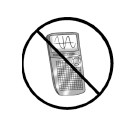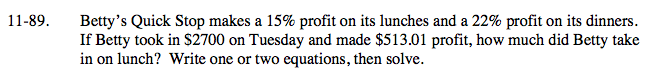### Home > A2C > Chapter Ch11 > Lesson 11.2.4 > Problem11-89

11-89.

Betty's Quick Stop makes a 15% profit on its lunches and a 22% profit on its dinners. If Betty took in $2700 on Tuesday and made$513.01 profit, how much did Betty take in on lunch? Write one or twoequations, then solve. Homework Help ✎Set up a system of equations.
Make x = lunch and y = dinner.

x + y = 2700
15x + 22y = 51,301

Set the equation like a mixture problem.

15x + 22(2700 − x) = 51,301

Distribute.

15x + 59,400 − 22x = 51,301

Solve for x.

7x = 8099

x = \$1157